## 5.1.2 Signal class estimation

26.4473GPPCodec for Enhanced Voice Services (EVS)Error concealment of lost packetsRelease 17TS

If possible, the class is directly derived from the coding mode in case of UC or VC modes, i.e. the class is UNVOICED_CLAS in case of UC frame and VOICED_CLAS in case of VC frame. Otherwise, it is estimated at the decoder as follows.

The frame classification at the decoder is based on the following parameters: zero-crossing parameter,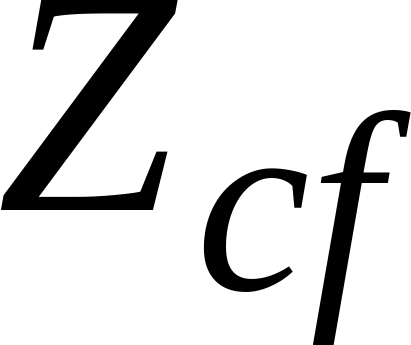, pitch-synchronous normalized correlation,, pitch coherence parameter,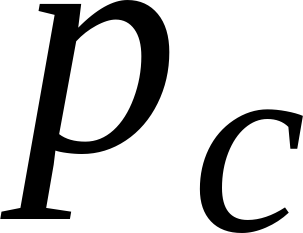, spectral tilt,, and pitch synchronous relative energy at the end of the frame,.

The zero-crossing parameter,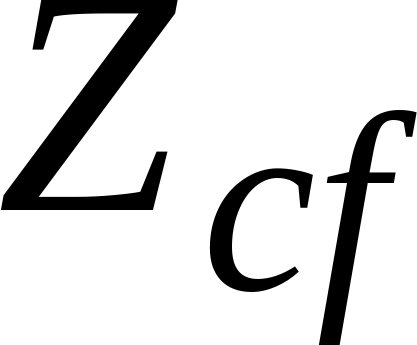, is averaged over the whole frame. That is,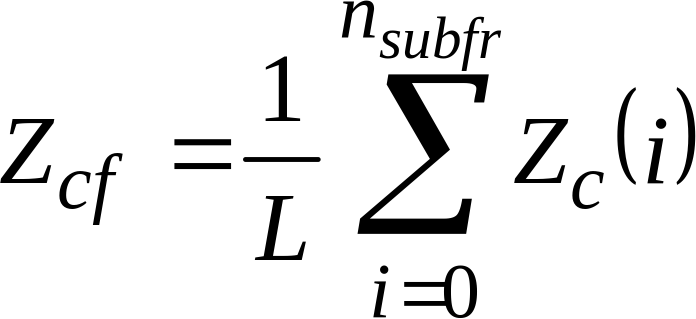(1)

where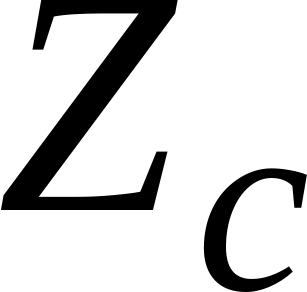is the number of times the signal sign of the synthesized signal,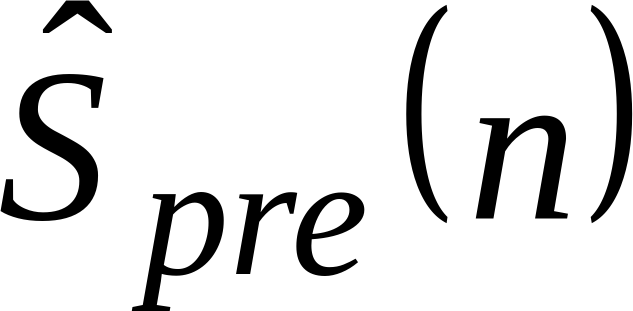, changes from positive to negative during subframe i. The number of subframes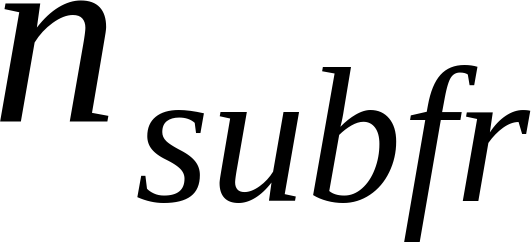depends of the internal sampling frequency which could be 12.8 kHz or 16 kHz. In case of 12.8kHz the number of subframes is 4 otherwise the number of subframes is 5. In case the internal sampling frequency is 16 kHz,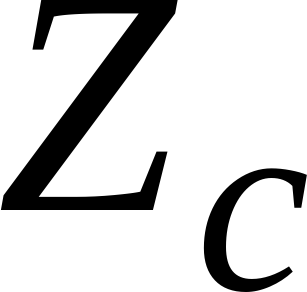is multiplied by 0.8.

The pitch synchronous normalized correlation is computed based on a pitch lag, T0, where T0 is the integer part of the pitch lag of the last subframe, or the average of the pitch lag of the last two subframe if it is larger than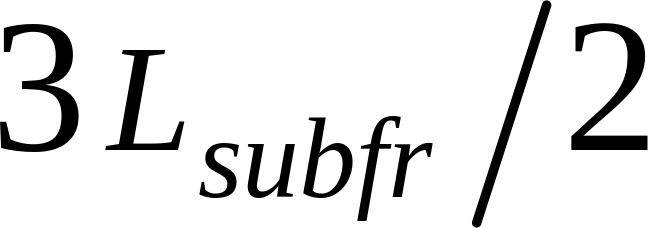, where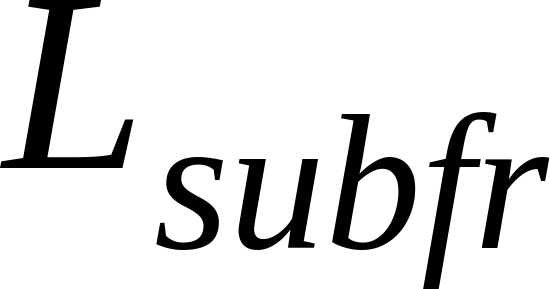= 64 is the subframe size. That is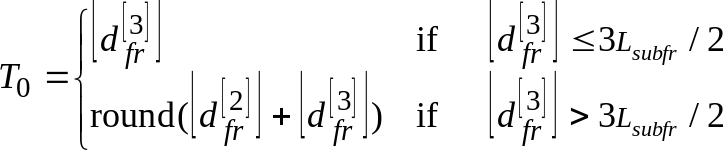(2)

where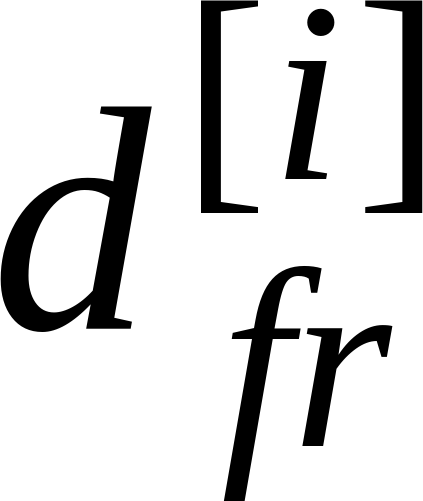is the fractional pitch lag at subframe i.

The pitch synchronous normalized correlation computed at the end of the frame is given by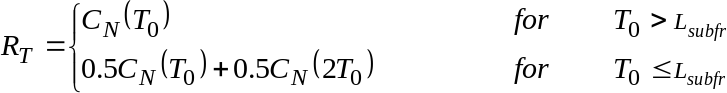(3)

where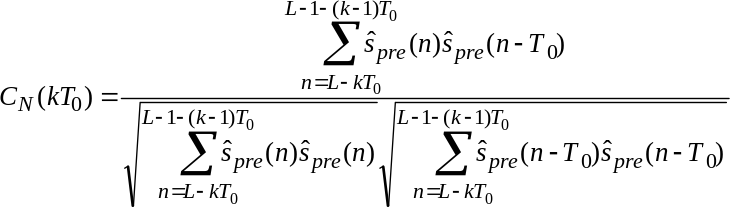(4)

where L is the frame size and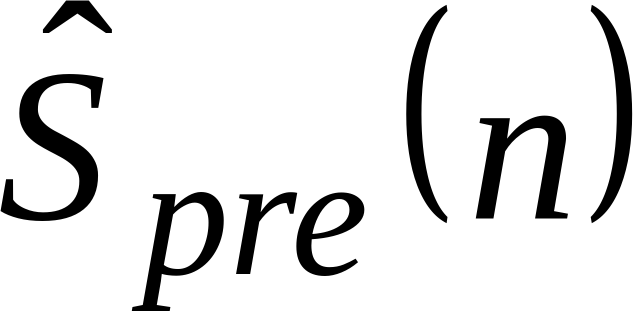is the synthesized speech signal.

The pitch coherence parameter is compute only in the case that the actual frame is not in TCX MDCT mode. The pitch coherence is given by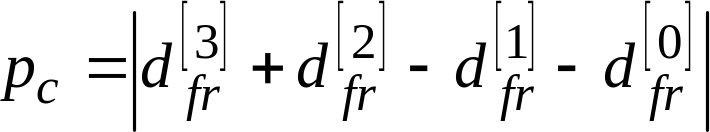(5)

where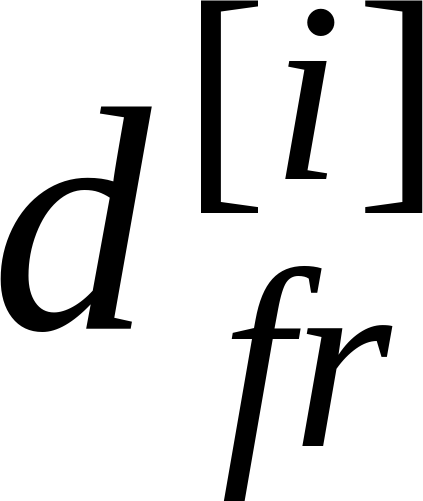is the fractional pitch lag at subframe i. In case the internal sampling frequency is 16 kHz,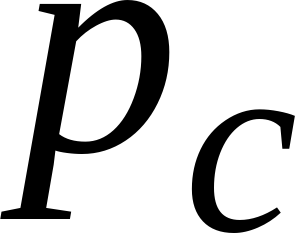is multiplied by 0.8.

The spectral tilt parameter,, is estimated based on the last 3 subframes and given by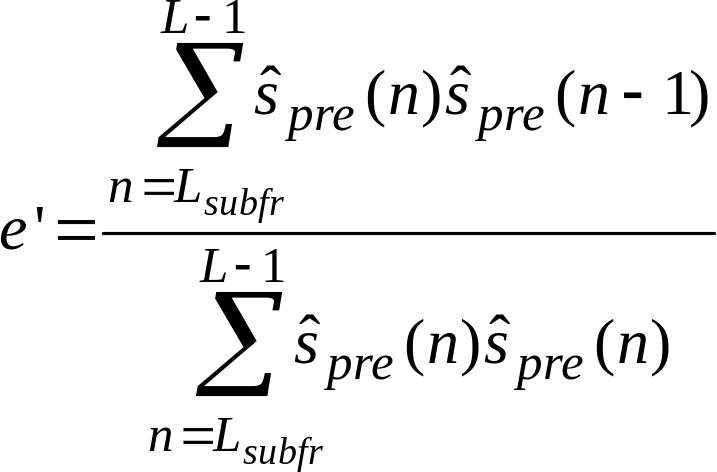(6)

The pitch synchronous relative energy at the end of the frame is given by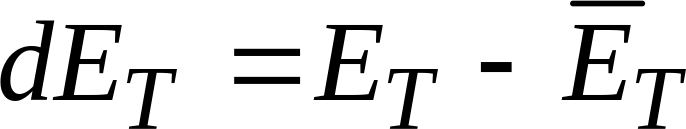(7)

where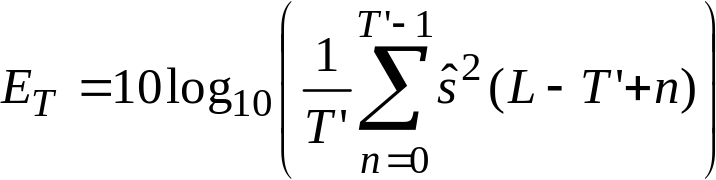(8)

and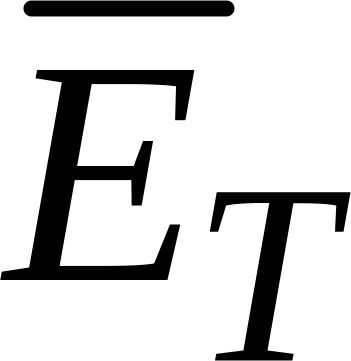is the long-term energy.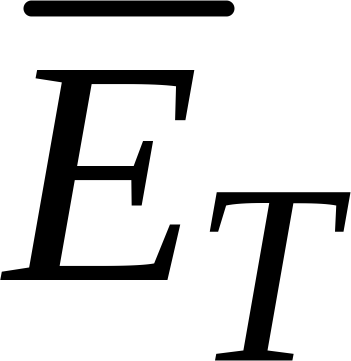is updated only when a current frame is classified as VOICED_CLAS and is of interoperable coding mode or isn’t of generic or transition coding mode, and is classified as VOICED_CLAS at the same time, using the relation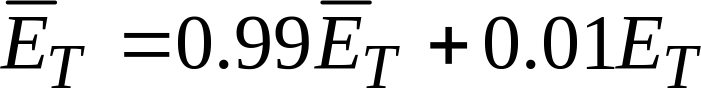(9)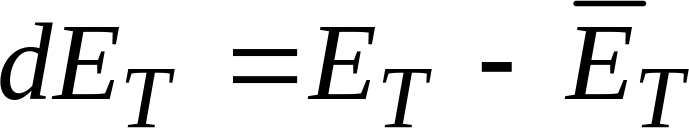(10)

The pitch lag value, T’, over which the energy,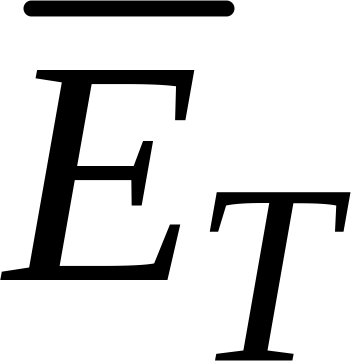, is computed is given by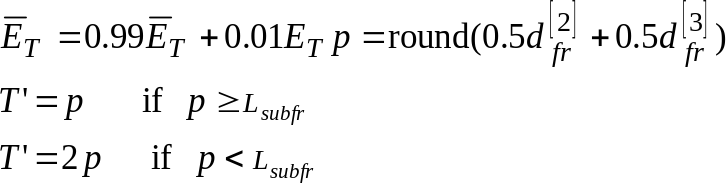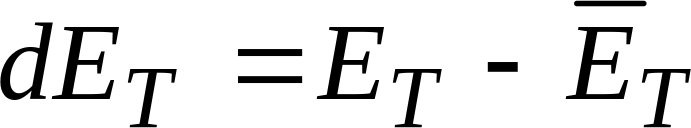(11)

To make the classification more robust, the classification parameters are considered together forming a function of merit,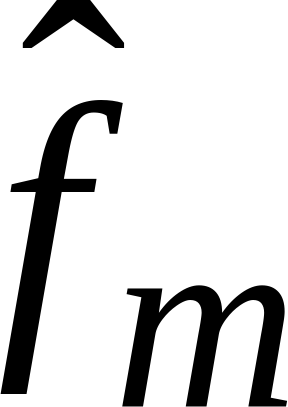. For that purpose, the classification parameters are first scaled so that each parameter’s typical value for unvoiced signal translates in 0 and each parameter’s typical value for voiced signal translates into 1. A linear function is used between them. The scaled version,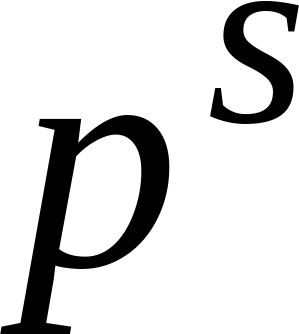, of a certain parameter,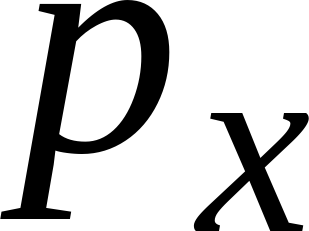, is obtained using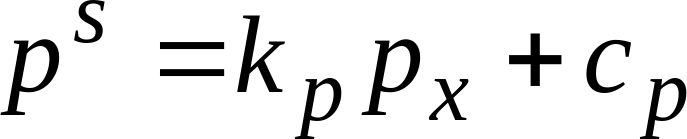(12)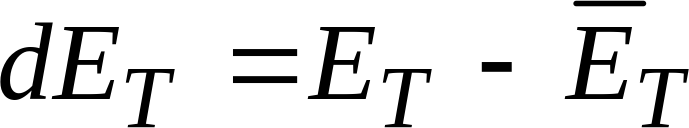(13)

and in case of pc the scaled parameter is constrained by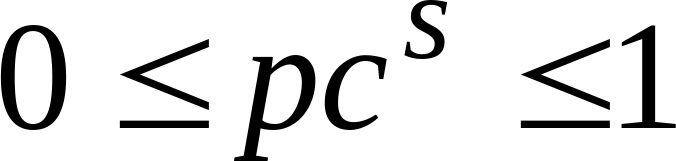.

The function coefficients,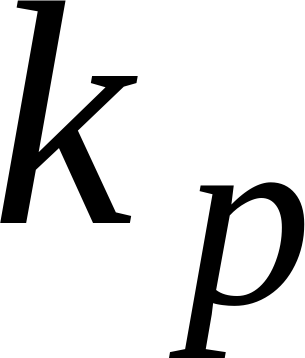, and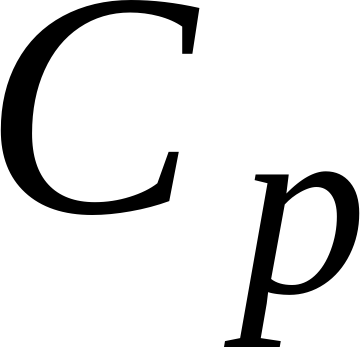, have been found experimentally for each of the parameters so that the signal distortion due to the concealment and recovery techniques used in the presence of frame erasures is minimal. The values used are summarized in Table 1 below.

Table 1: Signal classification parameters at the decoder

Parameter

Meaning

Kp

cpNormalized correlation

0.8547

0.2479Spectral tilt

0.8333

0.2917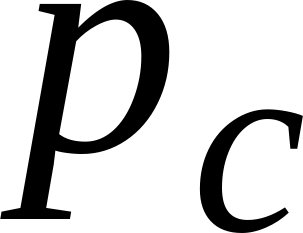Pitch coherence

–0.0357

1.6071Relative frame energy

0.04

0.56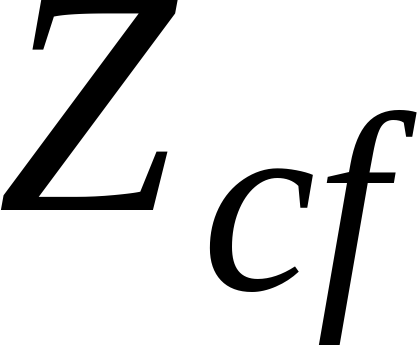Zero-crossing counter

–0.04

2.52

The merit function has been defined as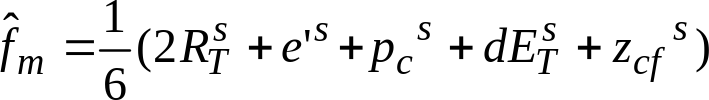(14)

where the superscript s indicates the scaled version of the parameters. In the case of 8-kHz sampled output and a decoded bit rate of 9.6kbps, the merit function, f, is further multiplied by 0.9.

In the case that the actual frame is not in TCX MDCT mode, the pitch coherence is not compute therefore the merit function has been defined as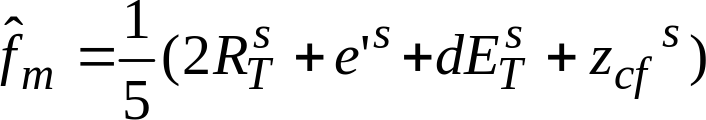(15)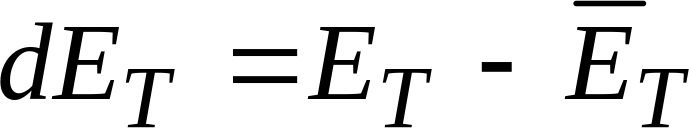(16)

The classification is performed using the merit function,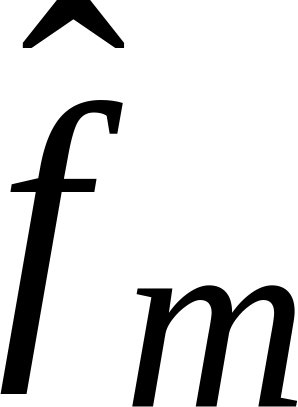, and following the rules summarized in Table 2. The default class is UNVOICED_CLAS. Note that the class ARTIFICIAL ONSET is set at the decoder if the frame follows an erased frame and artificial onset reconstruction is used as described in subclause 5.3.3.4.2.

Table 2: Signal classification rules at the decoder

Previous frame class

Rule

Current frame class

ONSET

ARTIFICIAL ONSET

VOICED_CLAS

VOICED TRANSITION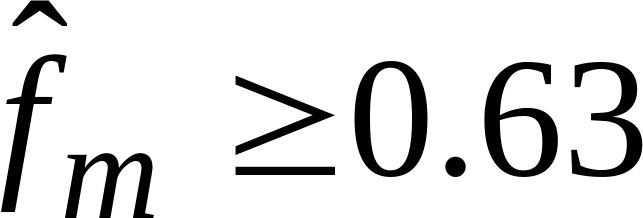VOICED_CLAS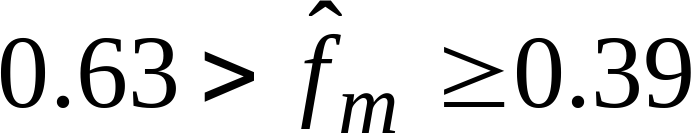VOICED TRANSITION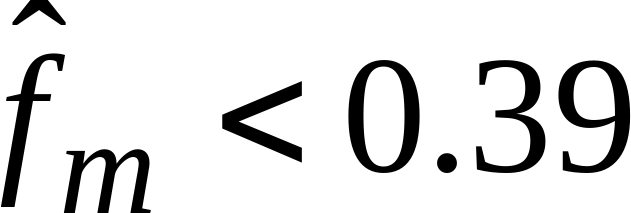UNVOICED_CLAS

UNVOICED TRANSITION

UNVOICED_CLAS

INACTIVE_CLAS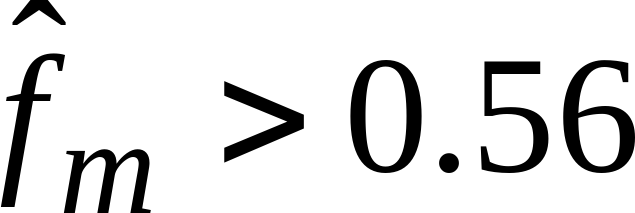ONSET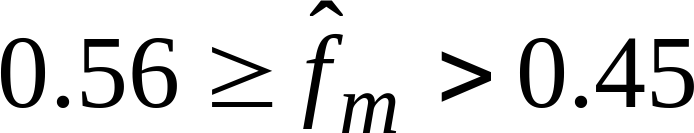UNVOICED TRANSITION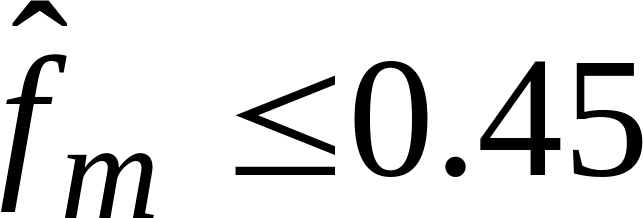UNVOICED_CLAS